MDC Corporation - Conversions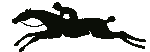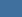Home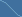ProductsHorse ShowExpert Witness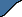Course Design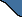Contacts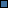Course DesignUSEF Distances Chart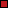Time Allowed Conversion FactorsFEI Distances (Feet/Meters)TIME CONVERSIONS
 USA / USEF 360 Yards/Min - Feet / 18.0 = Time Allowed 382 Yards/Min - Feet / 19.1 = Time Allowed

 FEI /CEF 300 Meters/Min - Feet / 16.4 or Meters / 5.00 325 Meters/Min - Feet / 17.8 or Meters / 5.40 350 Meters/Min - Feet / 19.1 or Meters / 5.83 375 Meters/Min - Feet / 20.5 or Meters / 6.25 400 Meters/Min - Feet / 21.9 or Meters / 6.66

 1 Meter = 39.37 Inches = 3.28 Feet 1 Foot = 12 Inches = 30.48 Centimeters Inches x .0254 = Meters 3 Inches = .076 Meters 6 inches = 0.15 Meters 5 cm = 2 Inches 10 cm = 4 Inches Feet x .3048 = Meters 1 Foot = 12 Inches 1 yard = 3 Feet

 Height - Width In Meters 1.10 = 3' 7" 1.40 = 4' 7" 1.70 = 5' 7" 2.00 = 6' 7" 1.15 = 3' 9" 1.45 = 4' 9" 1.75 = 5' 9" 2.05 = 6' 9" 1.20 = 3' 11" 1.50 = 4' 11" 1.80 = 5' 11" 2.10 = 6' 11" 1.25 = 4' 1" 1.55 = 5' 1" 1.85 = 6' 1" 2.15 = 7' 1" 1.30 = 4' 3" 1.60 = 5' 3" 1.90 = 6' 3" 2.20 = 7' 3" 1.35 = 4' 5" 1.65 = 5' 5" 1.95 = 6' 5" 2.25 = 7' 5"